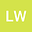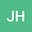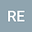loading page

Rational Solutions of Multi-component Nonlinear Schrödinger Equation and Complex Modified KdV Equation
•••• Lihong Wang,
• Jingsong He,
• Róbert Erdélyi
Lihong Wang
Ningbo University
Author ProfileJingsong He
Shenzhen University
Author ProfileRóbert Erdélyi
The University of Sheffield
Author Profile## Abstract

In this paper, the critical condition to achieve rational solutions of the multi-component nonlinear Schr\”odinger equation is proposed by introducing two nilpotent Lax matrices. Taking the series multisections of the vector eigenfunction as a set of fundamental eigenfunctions,an explicit formula of the $n$th-order rational solution is obtained by the degenerate Darboux transformation, which is used to generate some new patterns of rogue waves. A conjecture about the degree of the $n$th-order rogue waves is summarized. This conjecture also holds for rogue waves of the multi-component complex modified Korteweg-de Vries equation. Finally, the semi-rational solutions of the Manakov system are discussed.

#### Peer review status:UNDER REVIEW

21 Apr 2021Submitted to Mathematical Methods in the Applied Sciences
21 Apr 2021Assigned to Editor
21 Apr 2021Submission Checks Completed
30 Apr 2021Reviewer(s) Assigned